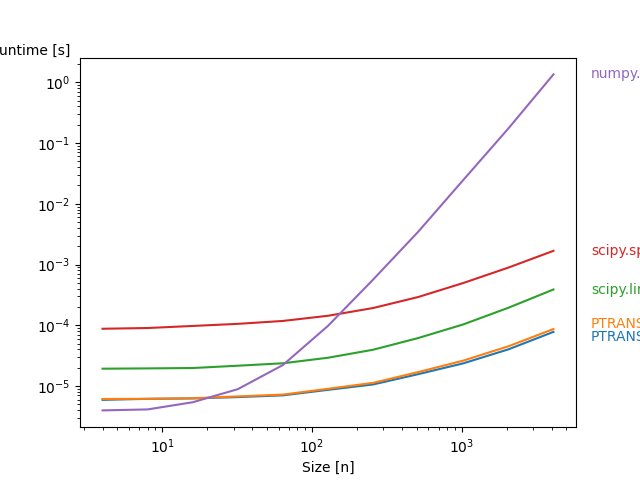# 4. Example: Performance for full matrices

Here we compare all algorithms for solving pentadiagonal systems provided by pentapy (except umf) using a full quadratic matrix as input.

To use this script you need to have the following packages installed:

• scipy

• perfplot

• matplotlib```Overall ━━━━━━━━━━━━━━━━━━━━━━━━━━━━━━━━━━━━━━━━ 100% 0:00:00
Kernels ━━━━━━━━━━━━━━━━━━━━━━━━━━━━━━━━━━━━━━━━ 100% 0:00:00
```

```import numpy as np
import perfplot

from pentapy import solve, tools

def get_les(size):
mat = (np.random.random((5, size)) - 0.5) * 1e-5
V = np.array(np.random.random(size) * 1e5)
M = tools.create_full(mat)
return M, V

def solve_1(in_val):
"""PTRANS-I"""
mat, V = in_val
return solve(mat, V, is_flat=False, solver=1)

def solve_2(in_val):
"""PTRANS-II"""
mat, V = in_val
return solve(mat, V, is_flat=False, solver=2)

def solve_3(in_val):
mat, V = in_val
return solve(mat, V, is_flat=False, solver=3)

def solve_4(in_val):
mat, V = in_val
return solve(mat, V, is_flat=False, solver=4)

def solve_5(in_val):
mat, V = in_val
return np.linalg.solve(mat, V)

perfplot.show(
setup=get_les,
kernels=[solve_1, solve_2, solve_3, solve_4, solve_5],
labels=[
"PTRANS-I",
"PTRANS-II",
"scipy.linalg.solve_banded",
"scipy.sparse.linalg.spsolve",
"numpy.linalg.solve",
],
n_range=[2**k for k in range(2, 13)],
xlabel="Size [n]",
logy=True,
)
```

Total running time of the script: ( 0 minutes 38.849 seconds)

Gallery generated by Sphinx-Gallery